### Rotating Gas Pipe (subsonic applications)

In the present section a rotating gas pipe with a varying cross section and friction is considered. Although the gas pipe Fanno is a special case of the rotating gas pipe, its governing equations constitute a singular limit to the equations presented here. Therefore, for a gas pipe without rotation and with constant cross section the equations here do not apply. The equivalent of Equation (74) now reads (, Table 10.2 on page 515):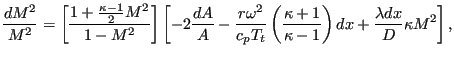(98)

where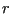is the shortest distance from the rotational axis,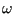is the rotational speed and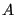is the local cross section of the pipe. Assuming that the radius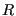of the pipe varies linearly along its length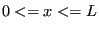: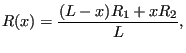(99)

one obtains for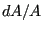: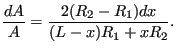(100)

Taking for,,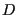and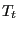the mean of their values at the end of the pipe one obtains for the second term in Equation (98)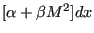where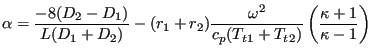(101)

and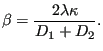(102)

Therefore, Equation (98) can now be written as: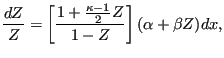(103)

or (using partial fractions):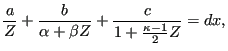(104)

where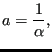(105)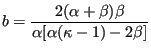(106)

and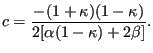(107)

From the above equations one notices that for a non-rotating pipe with constant cross section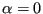and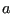and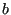become undeterminate. Therefore, although the gas pipe Fanno is a special case, the present formulas cannot be used for this element type. Integrating Equation (104) leads to: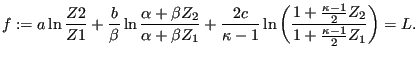(108)

Its derivatives are: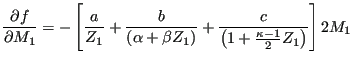(109)

and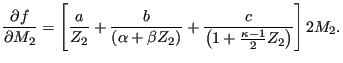(110)

Focussing on the subsonic range, one has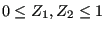. Therefore, the only term in Equation (108) which may cause problems is the second term. This is because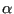and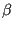do not necessarily have the same sign, therefore the logarithm may be undefined, i.e. the function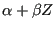may have a zero in between the ends of the pipe. This boils down to the condition (cf. Equation (98)) that in part of the element the Mach number is increasing and in part decreasing.

In general, convergence of a pipe and friction leads to increasing Mach numbers, divergence and centrifugal forces to decreasing Mach numbers. Sonic conditions should be avoided during the calculation. Especially if sonic conditions are observed at the end of a converged calculation, the result may not be correct.

Although the rotating pipe is adiabatic, i.e. no heat is transported to the envoronment, the total temperature changes due to conversion of rotational energy into heat or vice versa. Centrifugal motion leads to a total temperature increase, centripetal motion to a decrease. The change in total temperature amounts to :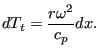(111)

For a linear varying radius integration leads to: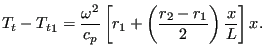(112)

Evaluating this expression for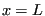yields the total temperature increase across the pipe. In order to estimate the total pressure increase (e.g. to arrive at sensible initial conditions) one can again use the formulas in  (discarding the friction effect):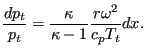(113)

Substituting a linear relationship forand the result just derived forleads to: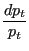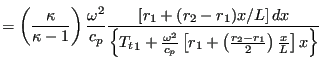(114)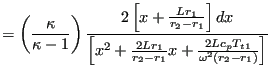(115)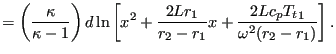(116)

Integrating finally leads to: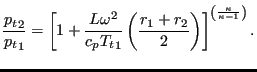(117)

It is important to notice that the rotating gas pipe is to be used in the relative (rotational) system (since the centrifugal force only exists in the rotational system). If used in the absolute system it has to be preceded by an absolute to relative element and followed by a relative to absolute element.

The rotating gas pipe is described by the following parameters (to be specified in that order on the line beneath the *FLUID SECTION, TYPE=ROTATING GAS PIPE card):

•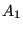: cross sectional area at node 1 (first node of element description)
•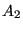: cross sectional area at node 2 (third node of element description)
•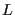: length of the element
•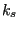: grain diameter at the pipe surface
• form factor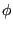•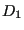: diameter at node 1; if the form factor is 1 the diameter is calculated form the area using the formula for a circle.
•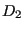: diameter at node 2; if the form factor is 1 the diameter is calculated form the area using the formula for a circle.
•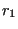: distance from the rotational axis of node 1.
•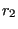: distance from the rotational axis of node 2.
•: rotational speed (in rad/s).

Example files: rotpipe1 up to rotpipe7.